# First Order Partial Differential Equations Method of characteristics

• Slides: 80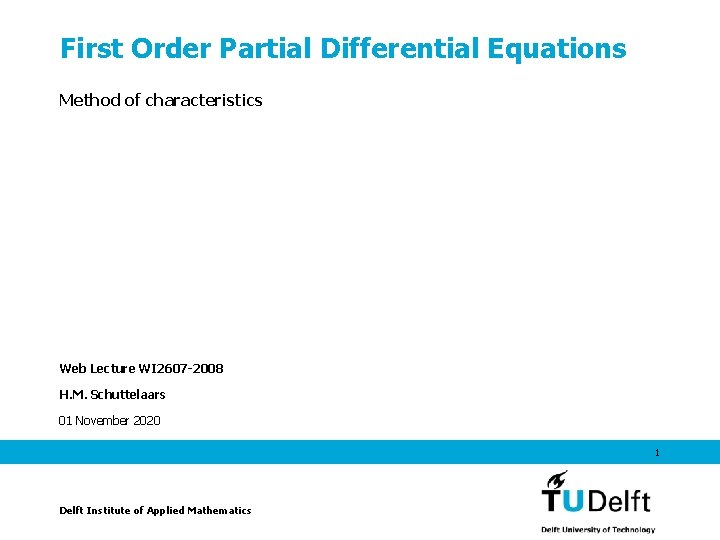First Order Partial Differential Equations Method of characteristics Web Lecture WI 2607 -2008 H. M. Schuttelaars 01 November 2020 1 Delft Institute of Applied Mathematics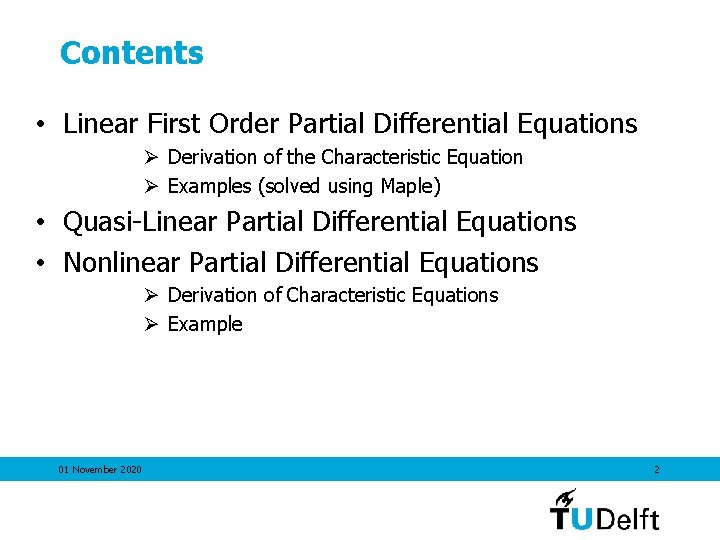Contents • Linear First Order Partial Differential Equations Ø Derivation of the Characteristic Equation Ø Examples (solved using Maple) • Quasi-Linear Partial Differential Equations • Nonlinear Partial Differential Equations Ø Derivation of Characteristic Equations Ø Example 01 November 2020 2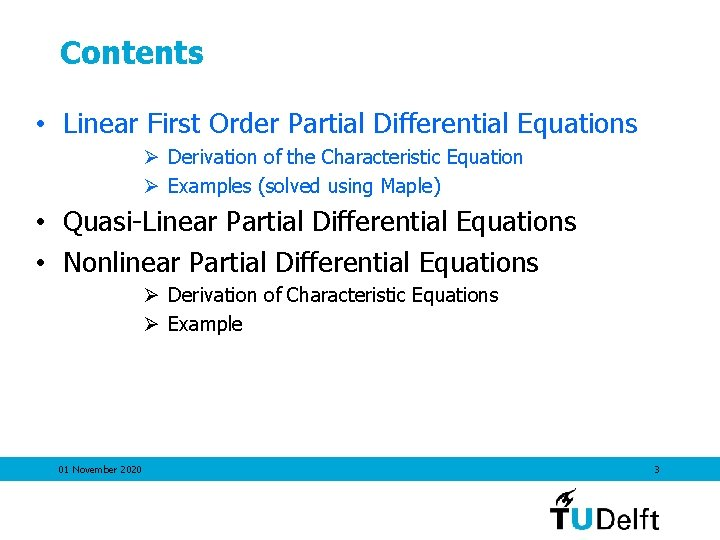Contents • Linear First Order Partial Differential Equations Ø Derivation of the Characteristic Equation Ø Examples (solved using Maple) • Quasi-Linear Partial Differential Equations • Nonlinear Partial Differential Equations Ø Derivation of Characteristic Equations Ø Example 01 November 2020 3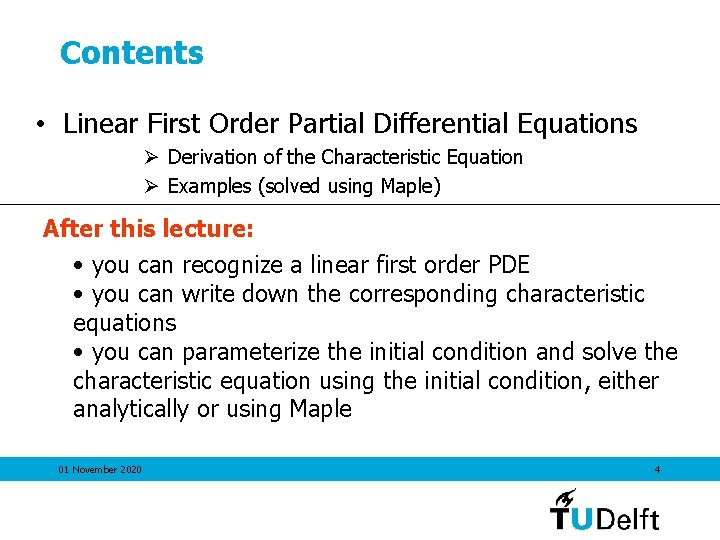Contents • Linear First Order Partial Differential Equations Ø Derivation of the Characteristic Equation Ø Examples (solved using Maple) After this lecture: • you can recognize a linear first order PDE • you can write down the corresponding characteristic equations • you can parameterize the initial condition and solve the characteristic equation using the initial condition, either analytically or using Maple 01 November 2020 4First Order Linear Partial Differential Equations Definition of a first order linear PDE: 01 November 2020 5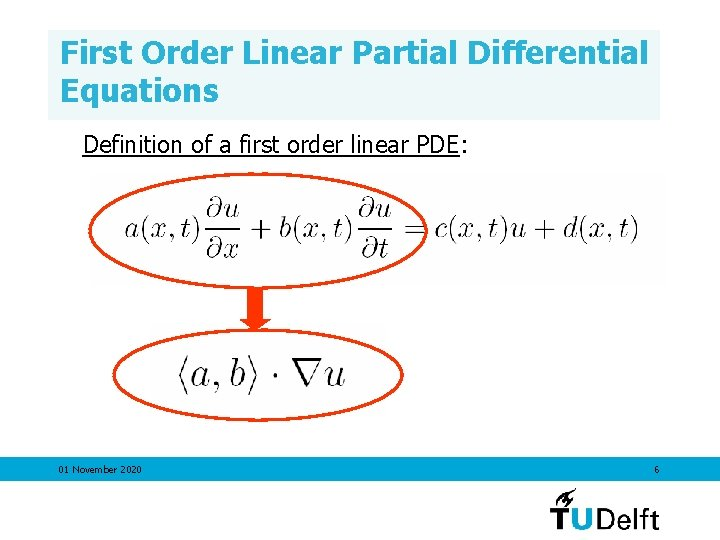First Order Linear Partial Differential Equations Definition of a first order linear PDE: 01 November 2020 6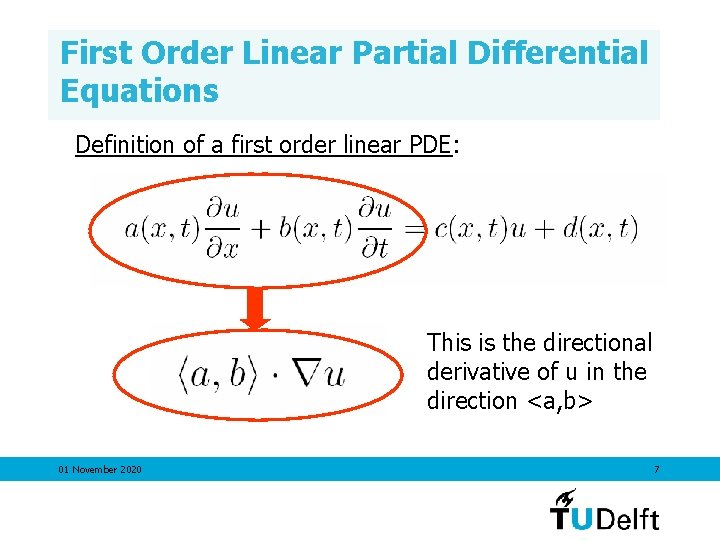First Order Linear Partial Differential Equations Definition of a first order linear PDE: This is the directional derivative of u in the direction <a, b> 01 November 2020 7First Order Linear Partial Differential Equations Example 01 November 2020 8First Order Linear Partial Differential Equations Plot the direction field: 01 November 2020 9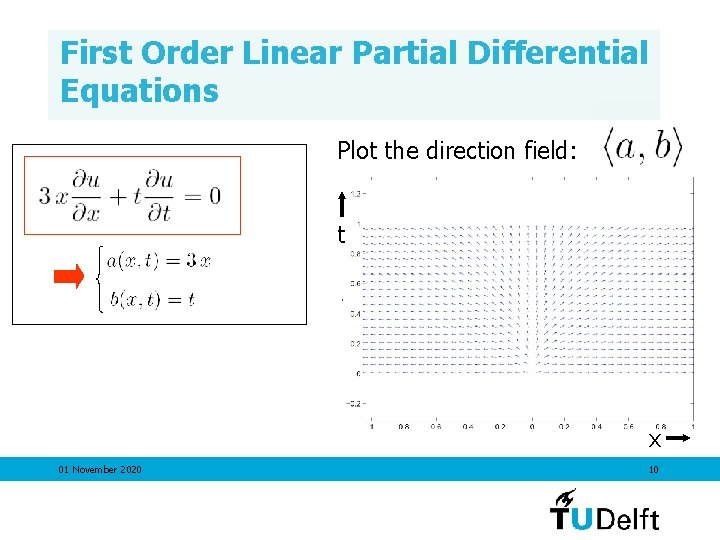First Order Linear Partial Differential Equations Plot the direction field: t x 01 November 2020 10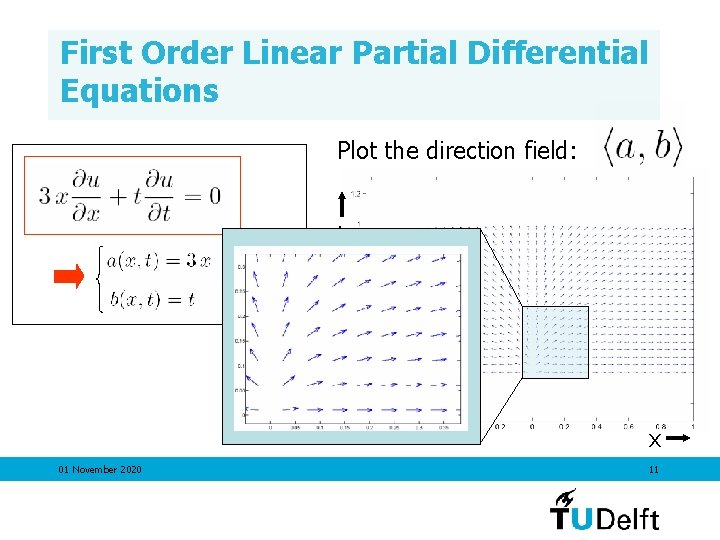First Order Linear Partial Differential Equations Plot the direction field: t x 01 November 2020 11First Order Linear Partial Differential Equations Direction field: Through every point, a curve exists that is tangent t to <a, b> everywhere. x 01 November 2020 12First Order Linear Partial Differential Equations Direction field: Through every point, a curve exists that is tangent to <a, b> everywhere: 1) Take points (0. 5, 0. 5), (-0. 1, 0. 5) and (0. 2, 0. 01) X X X 01 November 2020 13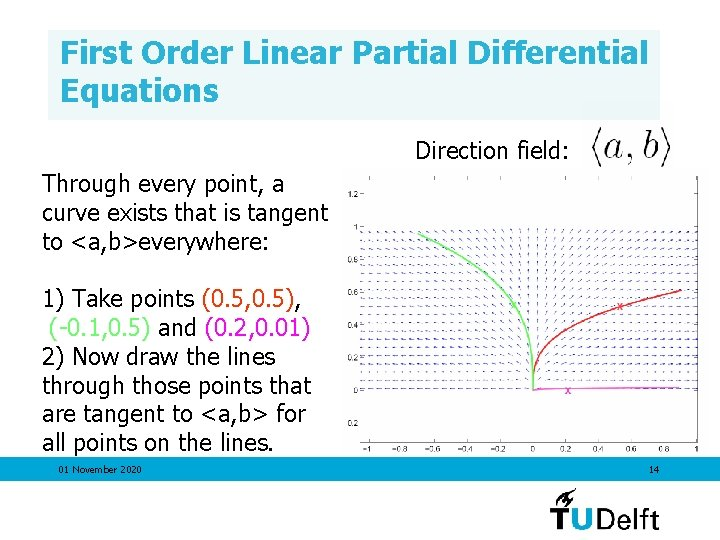First Order Linear Partial Differential Equations Direction field: Through every point, a curve exists that is tangent to <a, b>everywhere: 1) Take points (0. 5, 0. 5), (-0. 1, 0. 5) and (0. 2, 0. 01) 2) Now draw the lines through those points that are tangent to <a, b> for all points on the lines. 01 November 2020 14First Order Linear Partial Differential Equations Zooming in on the line through (0. 5, 0. 5), tangent to <a, b> for all x en t on the line: 01 November 2020 Direction field: 15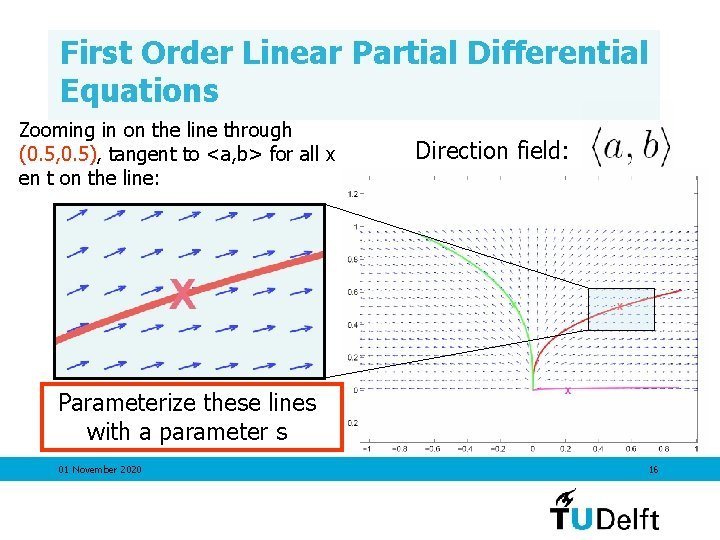First Order Linear Partial Differential Equations Zooming in on the line through (0. 5, 0. 5), tangent to <a, b> for all x en t on the line: Direction field: Parameterize these lines with a parameter s 01 November 2020 16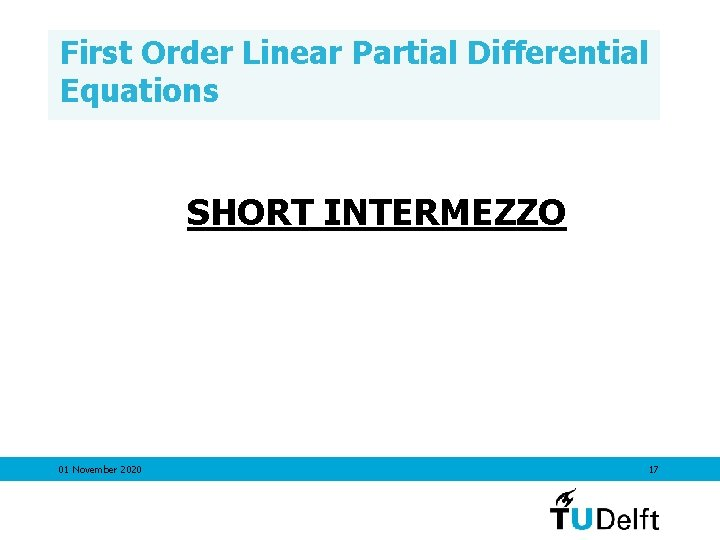First Order Linear Partial Differential Equations SHORT INTERMEZZO 01 November 2020 17First Order Linear Partial Differential Equations SHORT INTERMEZZO Parameterization of a line in 2 dimensions Parameter representation of a circle 01 November 2020 18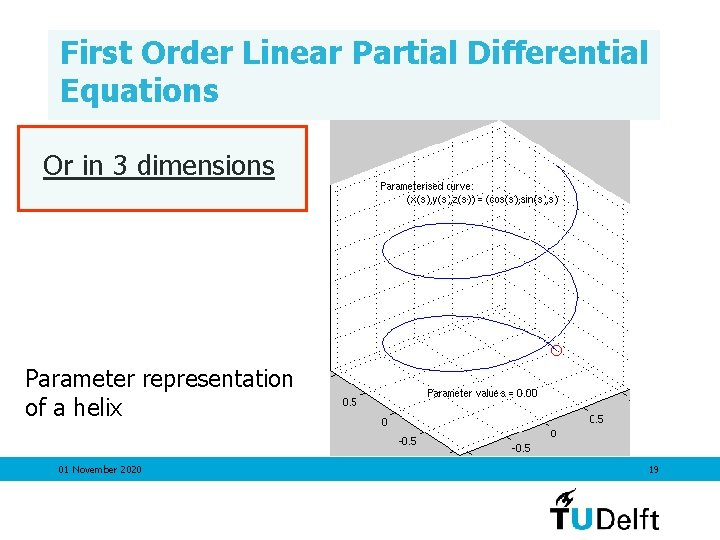First Order Linear Partial Differential Equations Or in 3 dimensions Parameter representation of a helix 01 November 2020 19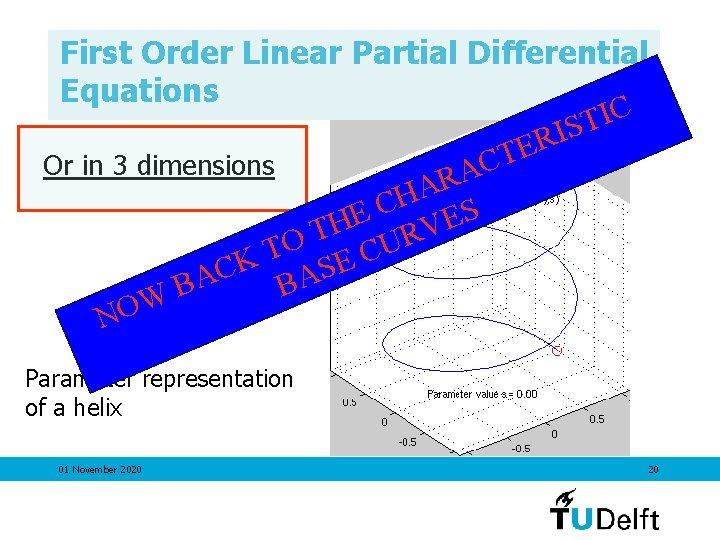First Order Linear Partial Differential Equations C I T S I R E T C Or in 3 dimensions A R A H S C E E H V T R O U T C K E S C A A B B W NO Parameter representation of a helix 01 November 2020 20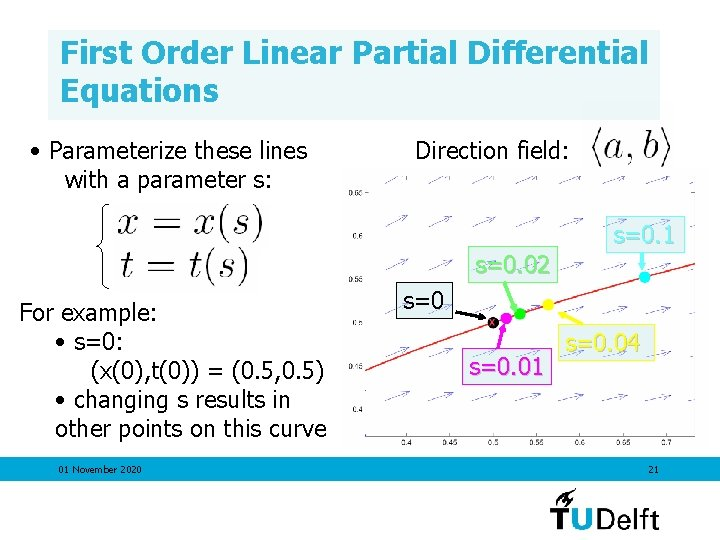First Order Linear Partial Differential Equations • Parameterize these lines with a parameter s: Direction field: s=0. 1 s=0. 02 For example: • s=0: (x(0), t(0)) = (0. 5, 0. 5) • changing s results in other points on this curve 01 November 2020 s=0. 01 s=0. 04 21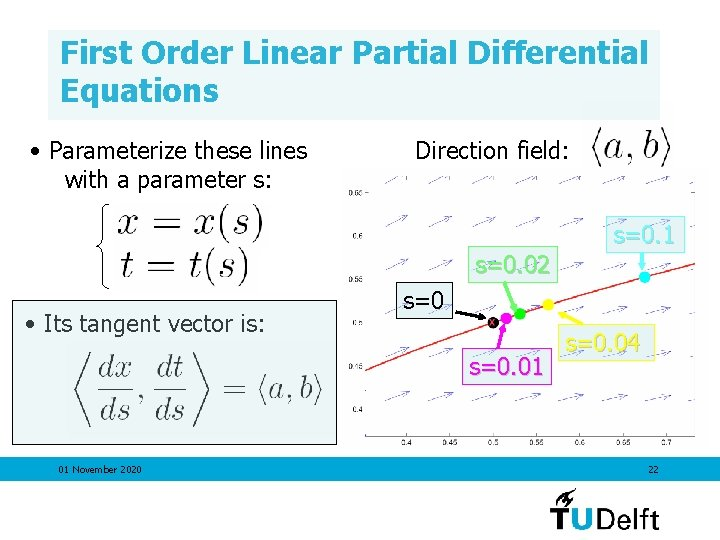First Order Linear Partial Differential Equations • Parameterize these lines with a parameter s: Direction field: s=0. 1 s=0. 02 • Its tangent vector is: s=0. 01 01 November 2020 s=0. 04 22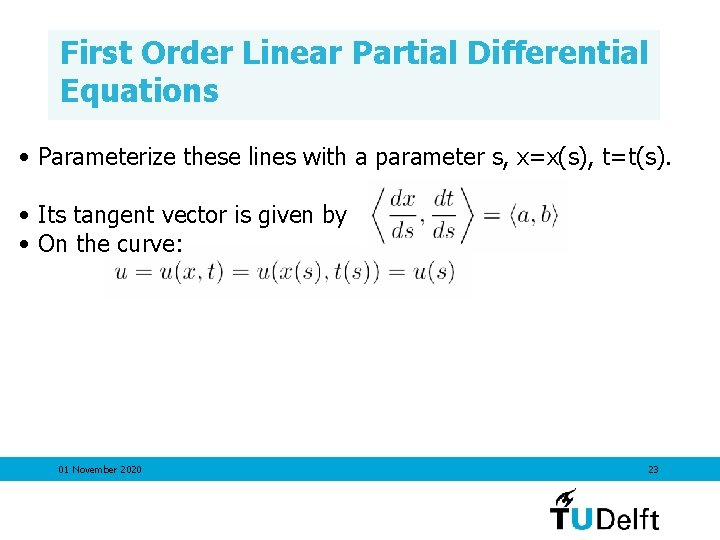First Order Linear Partial Differential Equations • Parameterize these lines with a parameter s, x=x(s), t=t(s). • Its tangent vector is given by • On the curve: 01 November 2020 23First Order Linear Partial Differential Equations • Parameterize these lines with a parameter s, x=x(s), t=t(s). • Its tangent vector is given by • On the curve: 01 November 2020 24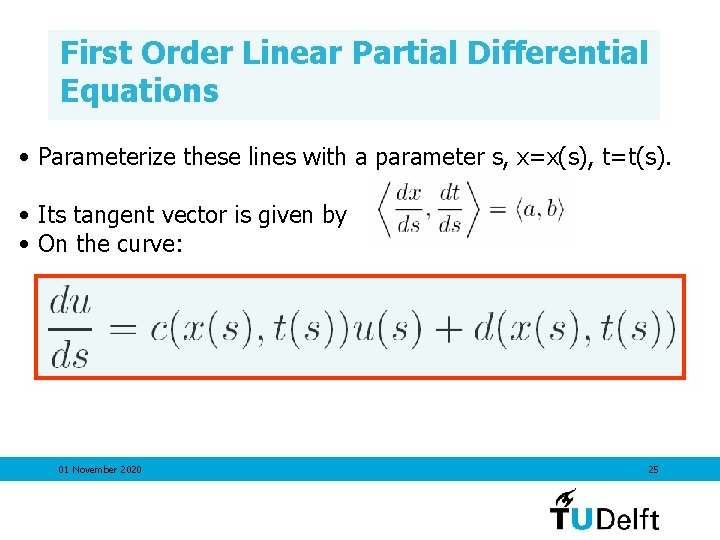First Order Linear Partial Differential Equations • Parameterize these lines with a parameter s, x=x(s), t=t(s). • Its tangent vector is given by • On the curve: 01 November 2020 25First Order Linear Partial Differential Equations • Its tangent vector is given by • On the curve: IN WORDS: THE PDE REDUCES TO AN ODE ON THE CHARACTERISTIC CURVES 01 November 2020 26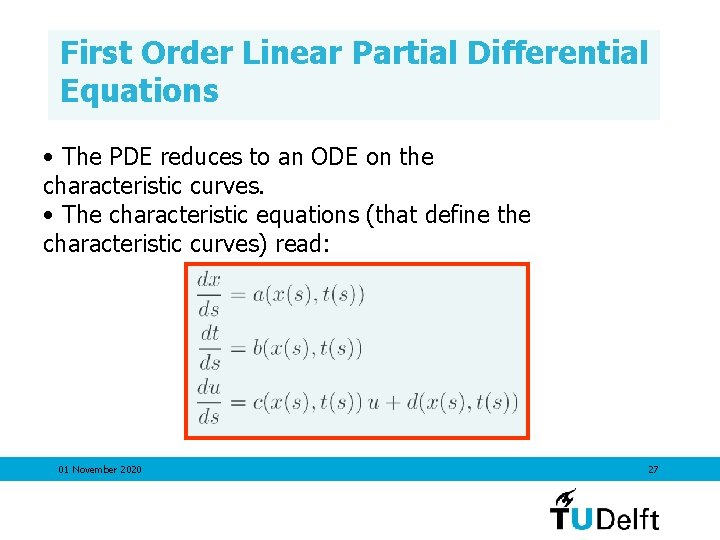First Order Linear Partial Differential Equations • The PDE reduces to an ODE on the characteristic curves. • The characteristic equations (that define the characteristic curves) read: 01 November 2020 27First Order Linear Partial Differential Equations • The PDE reduces to an ODE on the characteristic curves. • The characteristic equations (that define the characteristic curves) read: One can solve for x(s) and t(s) without solving for u(s). 01 November 2020 28First Order Linear Partial Differential Equations • The PDE reduces to an ODE on the characteristic curves. • The characteristic equations (that define the characteristic curves) read: One can solve for x(s) Gives the characteristic and t(s) without solving base curves for u(s). 01 November 2020 29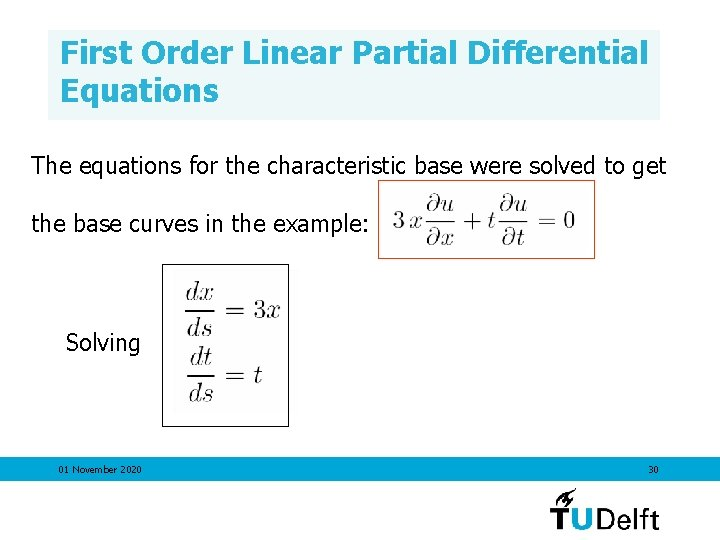First Order Linear Partial Differential Equations The equations for the characteristic base were solved to get the base curves in the example: Solving 01 November 2020 30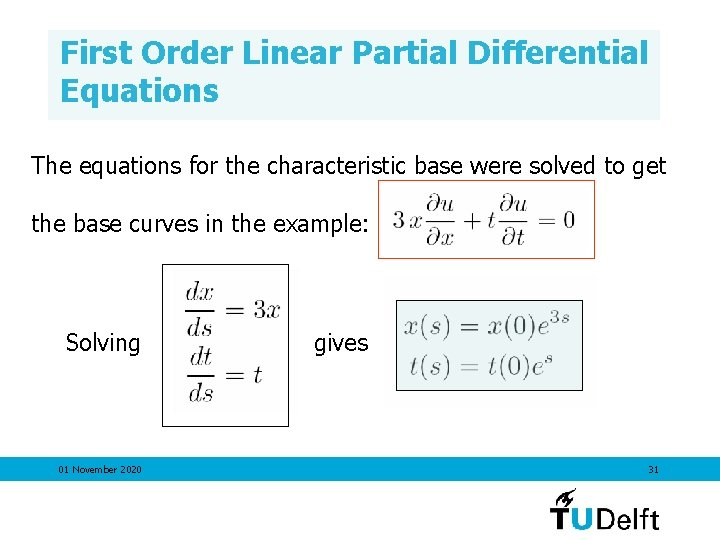First Order Linear Partial Differential Equations The equations for the characteristic base were solved to get the base curves in the example: Solving 01 November 2020 gives 31First Order Linear Partial Differential Equations This parameterisation, i. e. , was plotted for (0. 5, 0. 5) (x(0), t(0)) = (-0. 1, 0. 5) (0. 2, 0. 01) by varying s! 01 November 2020 32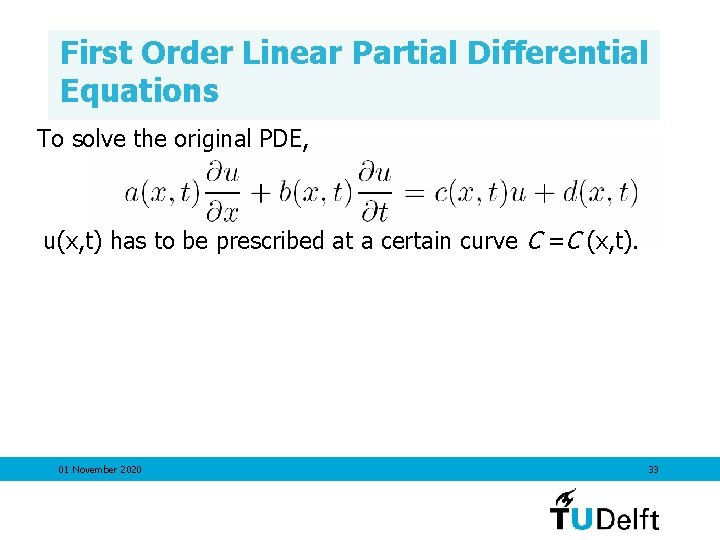First Order Linear Partial Differential Equations To solve the original PDE, u(x, t) has to be prescribed at a certain curve C =C (x, t). 01 November 2020 33First Order Linear Partial Differential Equations To solve the original PDE, u(x, t) has to be prescribed at a certain curve C =C (x, t). The corresponding system of ODE’s has to be solved such that u(x, t) has the prescribed value at this curve C. 01 November 2020 34First Order Linear Partial Differential Equations The corresponding system of ODE’s has to be solved such that u(x, t) has the prescribed value at this curve C. • As a first step, parameterize the initial curve C with the parameter τ: x=x(τ), t=t(τ) and u=u(τ). 01 November 2020 35First Order Linear Partial Differential Equations The corresponding system of ODE’s has to be solved such that u(x, t) has the prescribed value at this curve C. • As a first step, parameterize the initial curve C with the parameter τ: x=x(τ), t=t(τ) and u=u(τ). • Next, the family of characteristic curves, determined by the points on C , may be parameterized by x=x(s, τ), t=t(x, τ) and u=u(s, τ), with the initial conditions prescribed for s=0. 01 November 2020 36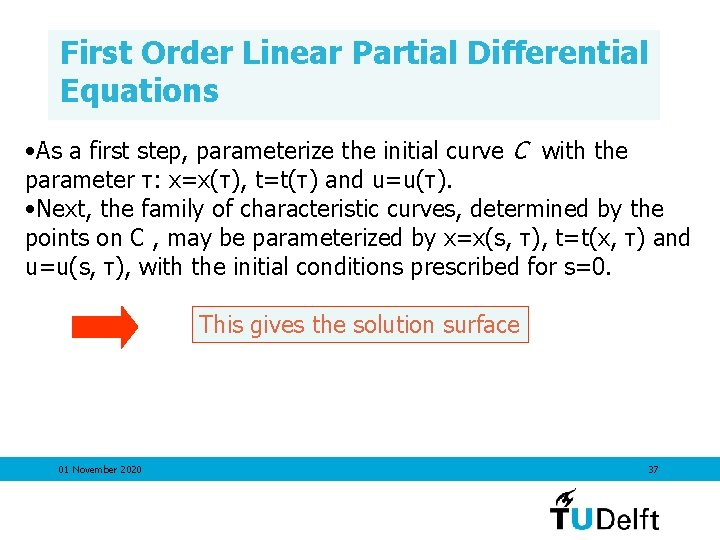First Order Linear Partial Differential Equations • As a first step, parameterize the initial curve C with the parameter τ: x=x(τ), t=t(τ) and u=u(τ). • Next, the family of characteristic curves, determined by the points on C , may be parameterized by x=x(s, τ), t=t(x, τ) and u=u(s, τ), with the initial conditions prescribed for s=0. This gives the solution surface 01 November 2020 37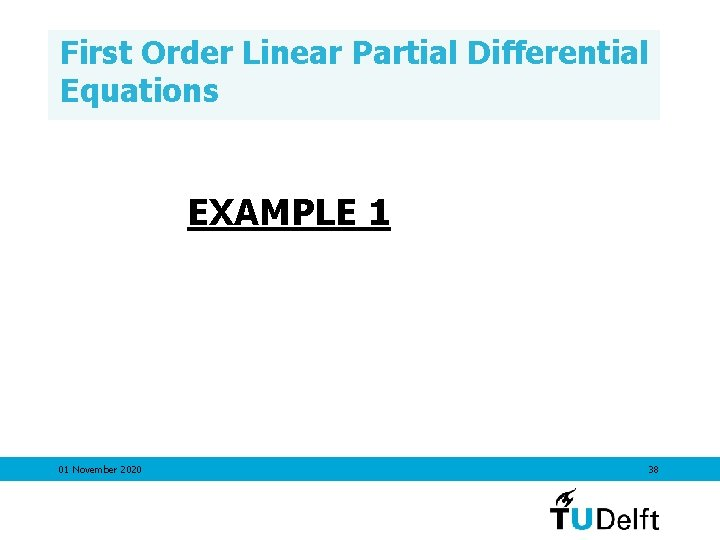First Order Linear Partial Differential Equations EXAMPLE 1 01 November 2020 38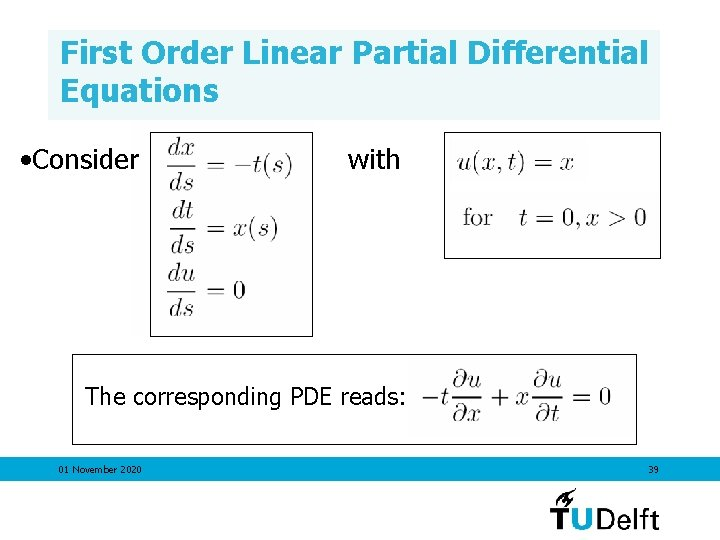First Order Linear Partial Differential Equations • Consider with The corresponding PDE reads: 01 November 2020 39First Order Linear Partial Differential Equations • Consider with • Parameterize this initial curve with parameter l: 01 November 2020 40First Order Linear Partial Differential Equations • Consider with • Parameterize this initial curve with parameter l: • Solve the characteristic equations with these initial conditions. 01 November 2020 41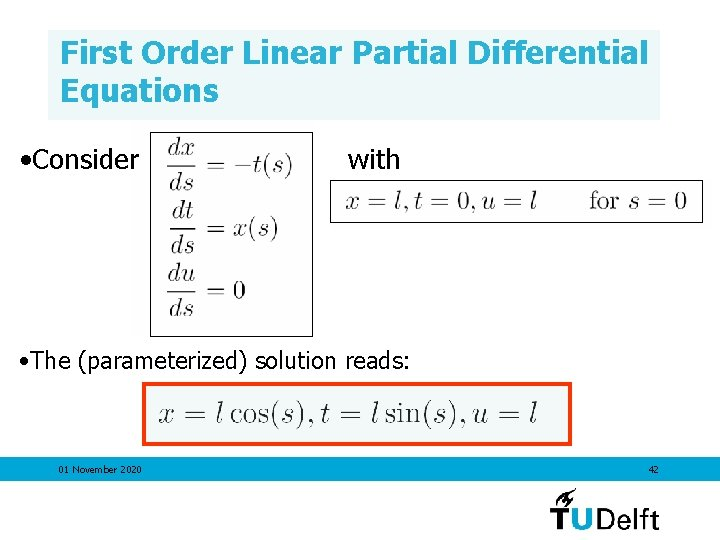First Order Linear Partial Differential Equations • Consider with • The (parameterized) solution reads: 01 November 2020 42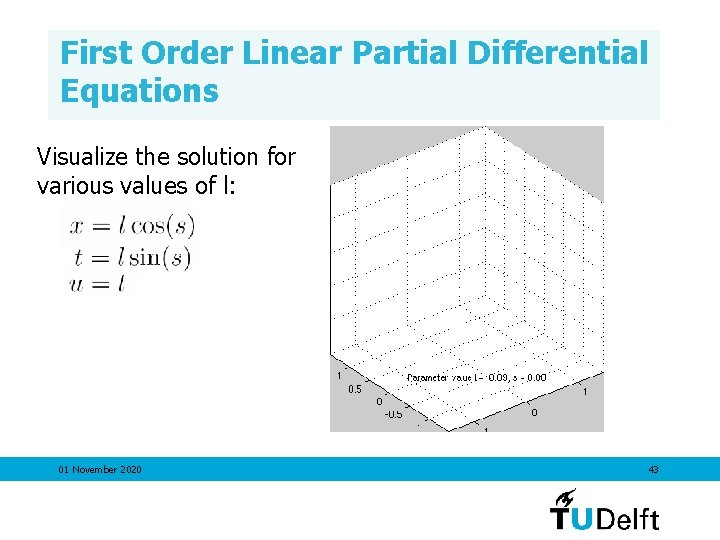First Order Linear Partial Differential Equations Visualize the solution for various values of l: 01 November 2020 43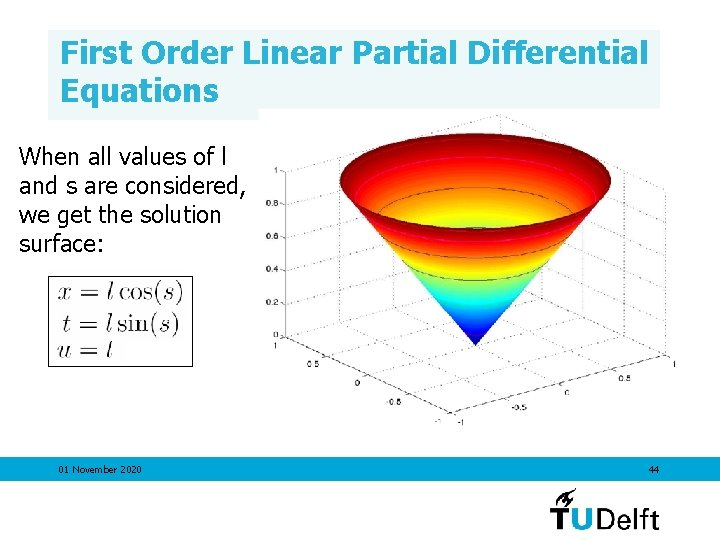First Order Linear Partial Differential Equations When all values of l and s are considered, we get the solution surface: 01 November 2020 44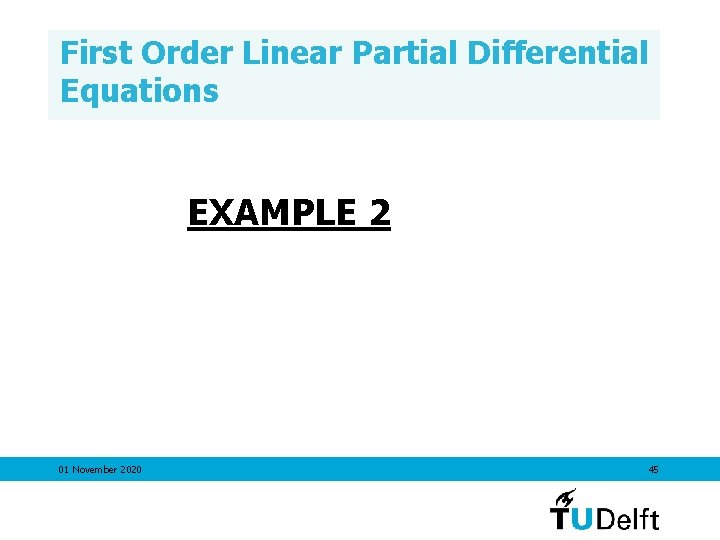First Order Linear Partial Differential Equations EXAMPLE 2 01 November 2020 45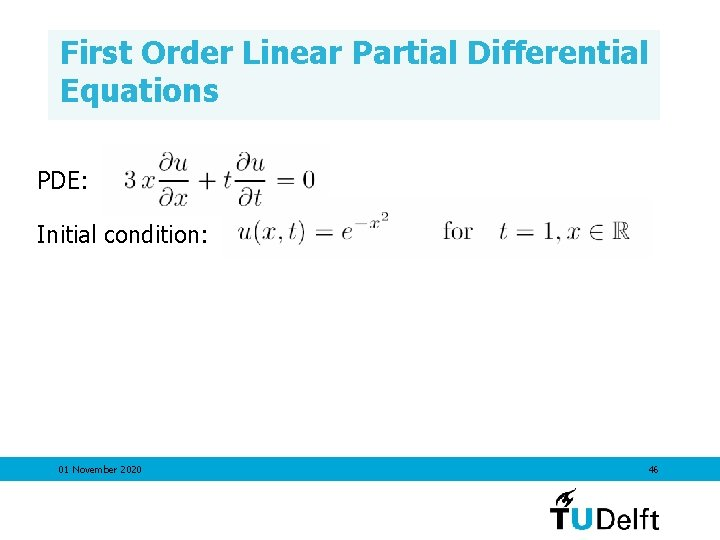First Order Linear Partial Differential Equations PDE: Initial condition: 01 November 2020 46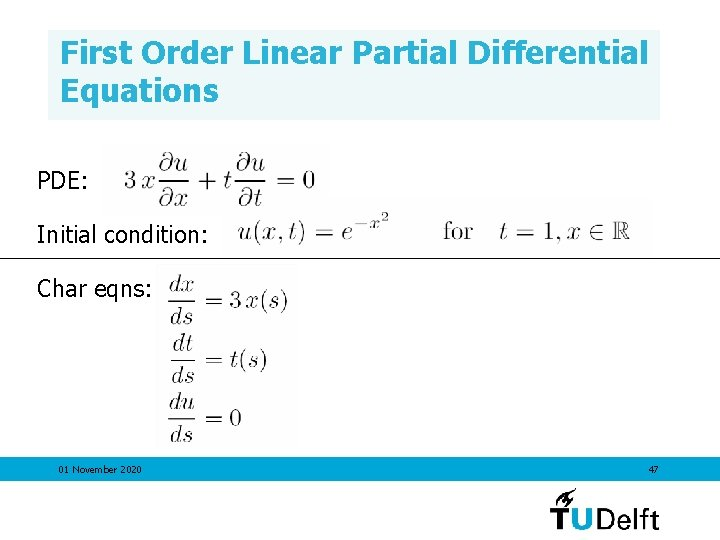First Order Linear Partial Differential Equations PDE: Initial condition: Char eqns: 01 November 2020 47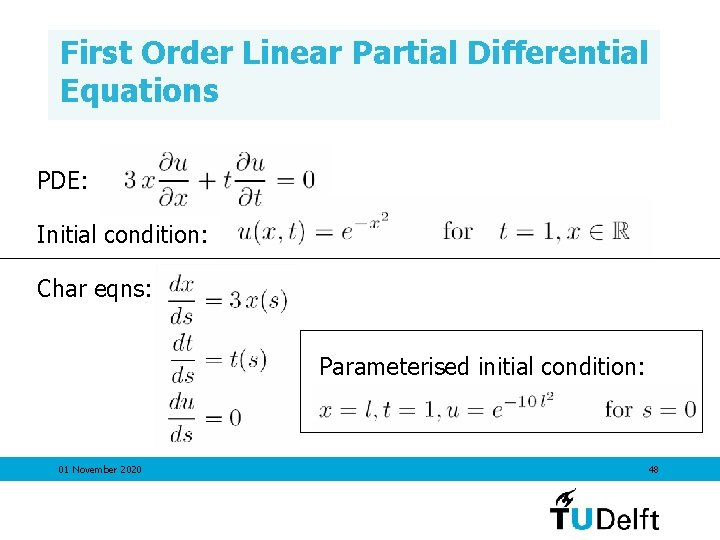First Order Linear Partial Differential Equations PDE: Initial condition: Char eqns: Parameterised initial condition: 01 November 2020 48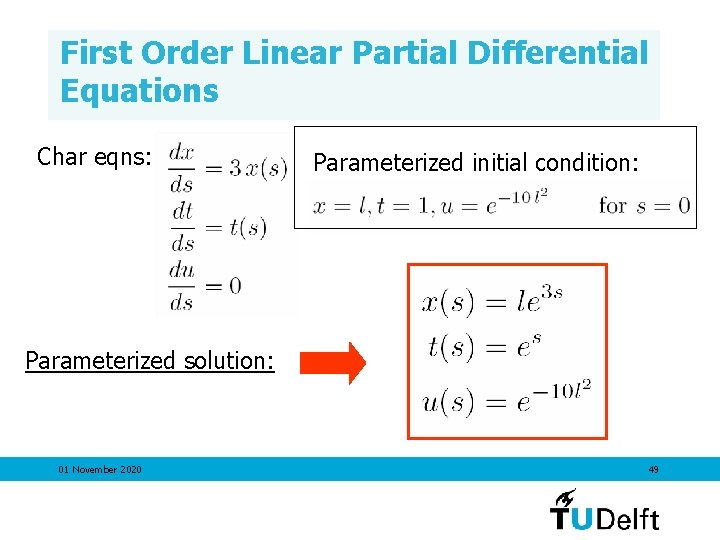First Order Linear Partial Differential Equations Char eqns: Parameterized initial condition: Parameterized solution: 01 November 2020 49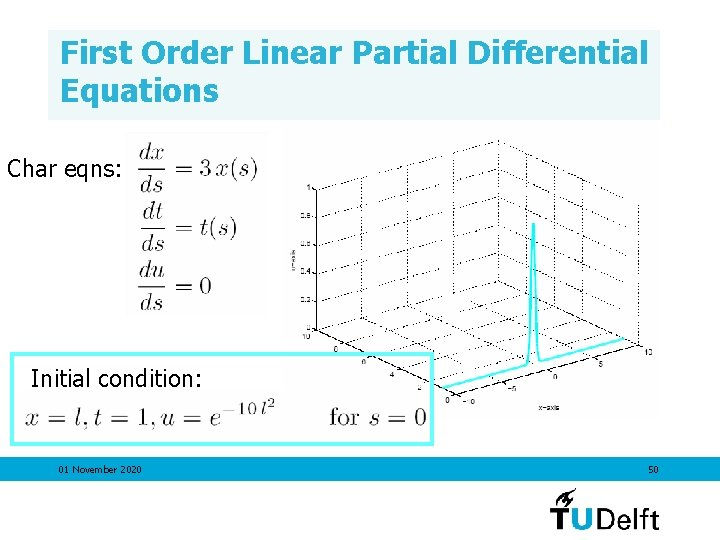First Order Linear Partial Differential Equations Char eqns: Initial condition: 01 November 2020 50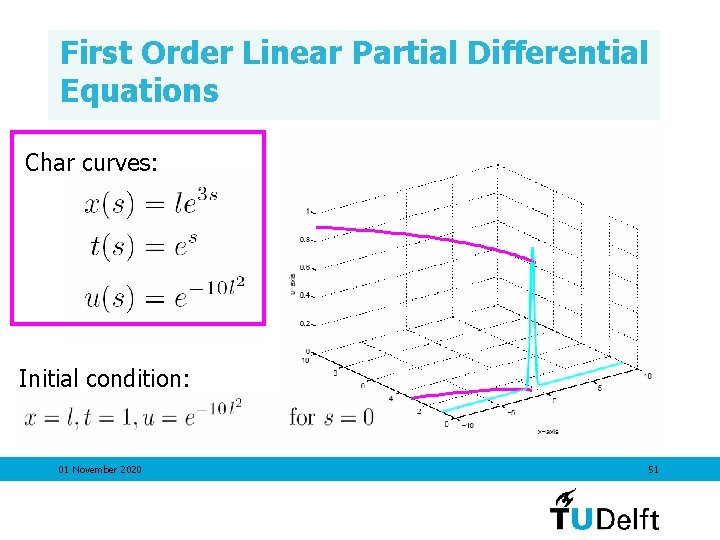First Order Linear Partial Differential Equations Char curves: Initial condition: 01 November 2020 51First Order Linear Partial Differential Equations This gives the solution surface, parameterised by s and l. 01 November 2020 52First Order Linear Partial Differential Equations This gives the solution surface, parameterised by s and l. This is the solution of the original PDE With initial condition 01 November 2020 53First Order Linear Partial Differential Equations Recipe to solve the PDE 1. To solve the partial differential equation explicitly, u(x, t) must be given at a certain curve C. As a first step, parameterize this curve with parameter l. 01 November 2020 54First Order Linear Partial Differential Equations 2. Write down the characteristic equations, note that x, t and u now depend on both the parameters s and l. 01 November 2020 55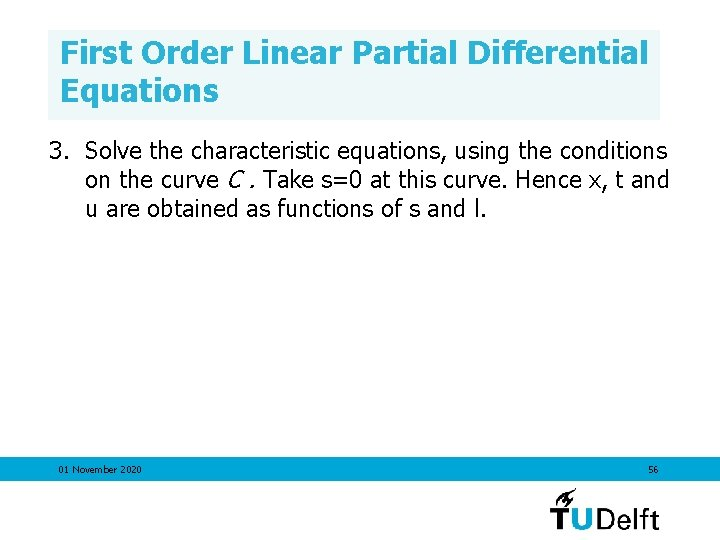First Order Linear Partial Differential Equations 3. Solve the characteristic equations, using the conditions on the curve C. Take s=0 at this curve. Hence x, t and u are obtained as functions of s and l. 01 November 2020 56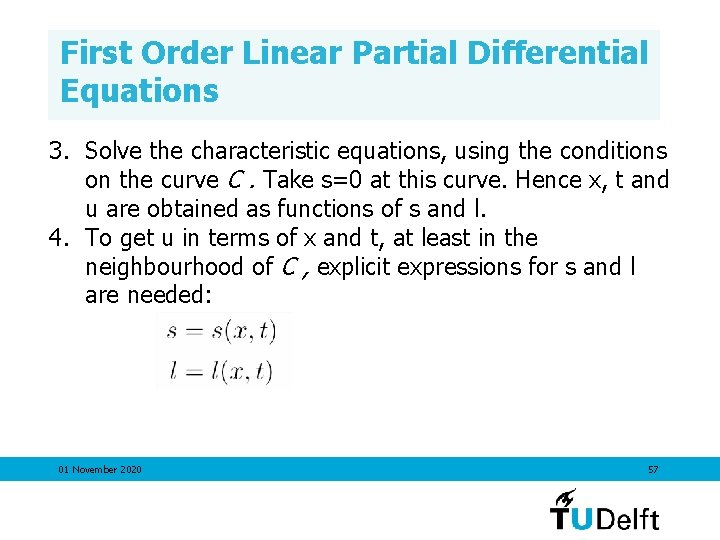First Order Linear Partial Differential Equations 3. Solve the characteristic equations, using the conditions on the curve C. Take s=0 at this curve. Hence x, t and u are obtained as functions of s and l. 4. To get u in terms of x and t, at least in the neighbourhood of C , explicit expressions for s and l are needed: 01 November 2020 57First Order Linear Partial Differential Equations 3. Solve the characteristic equations, using the conditions on the curve C. Take s=0 at this curve. Hence x, t and u are obtained as functions of s and l. 4. To get u in terms of x and t, at least in the neighbourhood of C , explicit expressions for s and l are needed: Implicit Function Theorem: possible in a neighborhood of C if 01 November 2020 58First Order Linear Partial Differential Equations 3. Solve the characteristic equations, using the conditions on the curve C. Take s=0 at this curve. Hence x, t and u are obtained as functions of s and l. 4. To get u in terms of x and t, at least in the neighbourhood of C , explicit expressions for s and l are needed: (Implicit Function Theorem) • possible in a neighborhood of C if • otherwise: no solution of infinitely many solutions 01 November 2020 59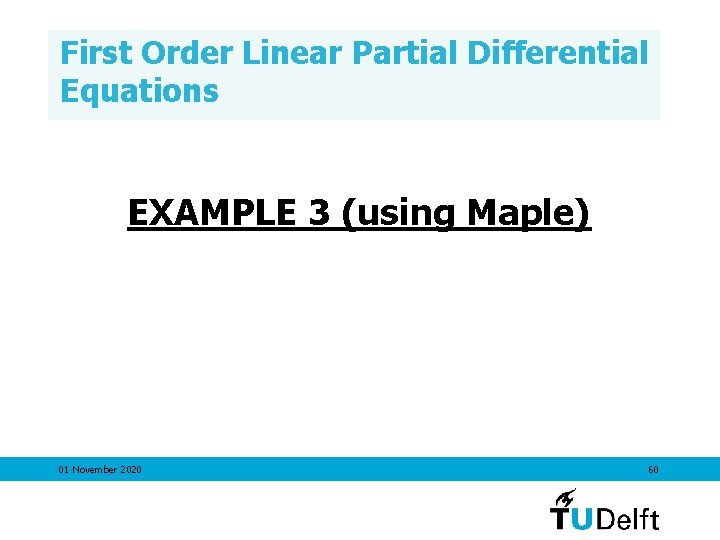First Order Linear Partial Differential Equations EXAMPLE 3 (using Maple) 01 November 2020 60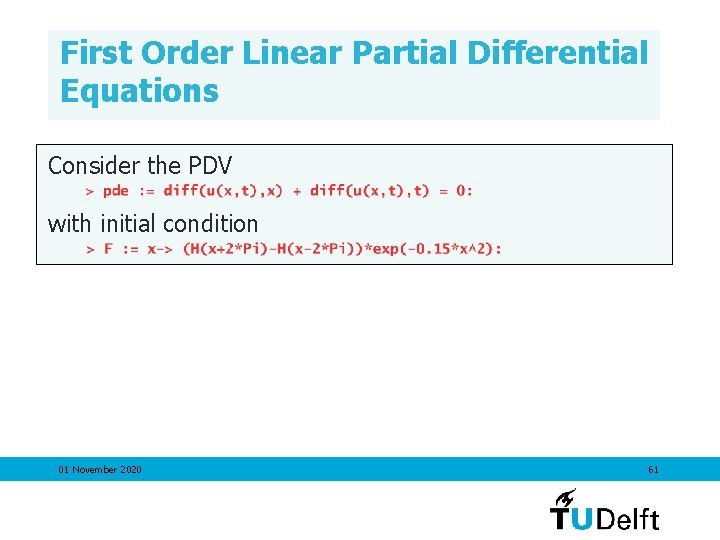First Order Linear Partial Differential Equations Consider the PDV with initial condition 01 November 2020 61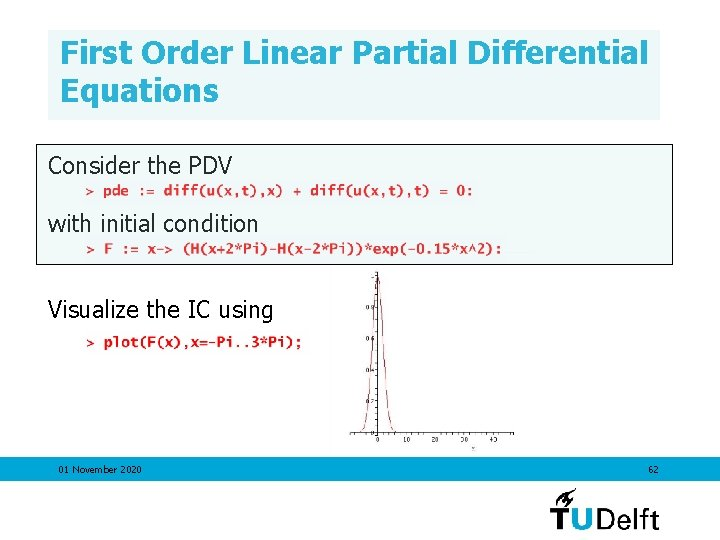First Order Linear Partial Differential Equations Consider the PDV with initial condition Visualize the IC using 01 November 2020 62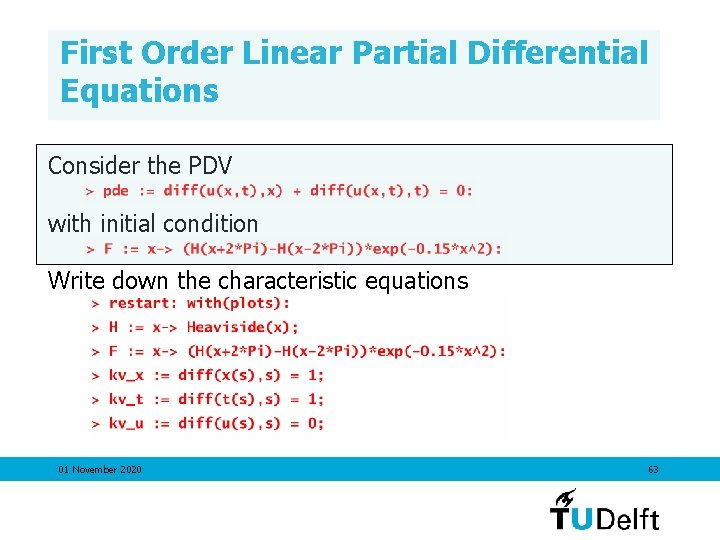First Order Linear Partial Differential Equations Consider the PDV with initial condition Write down the characteristic equations 01 November 2020 63First Order Linear Partial Differential Equations Consider the PDV with initial condition Solve the characteristic equations using dsolve: 01 November 2020 64First Order Linear Partial Differential Equations Consider the PDV with initial condition Solve the characteristic equations using dsolve: 01 November 2020 65First Order Linear Partial Differential Equations Use the solution sol to vizualize the solution surface: Note that in this example we can easily find s and l in terms of x and t and hence get the solution in terms of x and t: s=t l = x-t 01 November 2020 66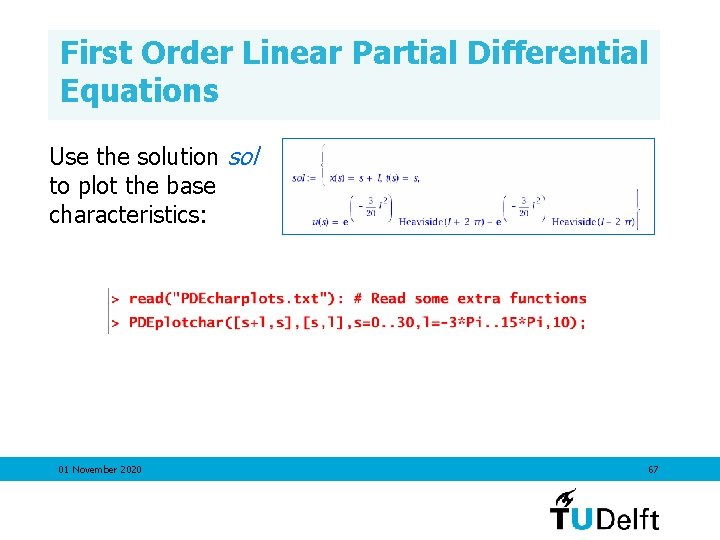First Order Linear Partial Differential Equations Use the solution sol to plot the base characteristics: 01 November 2020 67First Order Linear Partial Differential Equations Use the solution sol to plot the base characteristics: 01 November 2020 68First Order Linear Partial Differential Equations Use the solution sol to vizualize the solution surface: 01 November 2020 69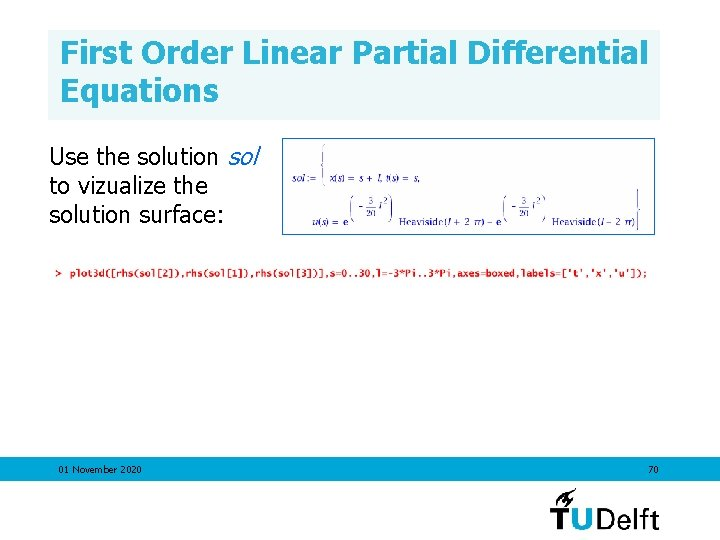First Order Linear Partial Differential Equations Use the solution sol to vizualize the solution surface: 01 November 2020 70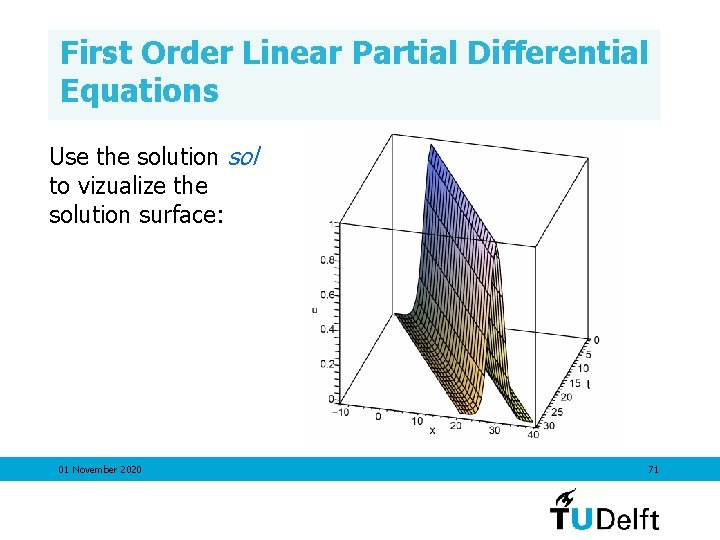First Order Linear Partial Differential Equations Use the solution sol to vizualize the solution surface: 01 November 2020 71First Order Linear Partial Differential Equations Use the solution sol to animate the solution in time: 01 November 2020 72First Order Linear Partial Differential Equations Use the solution sol to animate the solution in time: 01 November 2020 73First Order Linear Partial Differential Equations EXAMPLE 4 (using Maple) 01 November 2020 74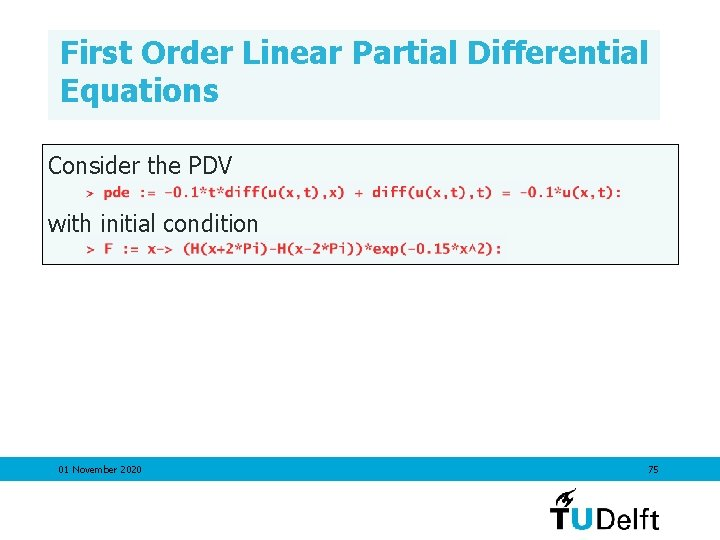First Order Linear Partial Differential Equations Consider the PDV with initial condition 01 November 2020 75First Order Linear Partial Differential Equations Consider the PDV with initial condition Write down the characteristic equations 01 November 2020 76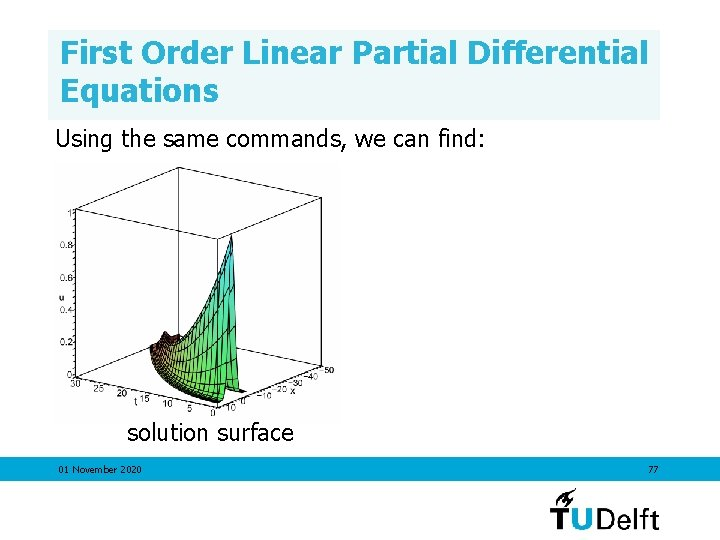First Order Linear Partial Differential Equations Using the same commands, we can find: solution surface 01 November 2020 77First Order Linear Partial Differential Equations Using the same commands, we can find: solution surface 01 November 2020 animation of the solution 78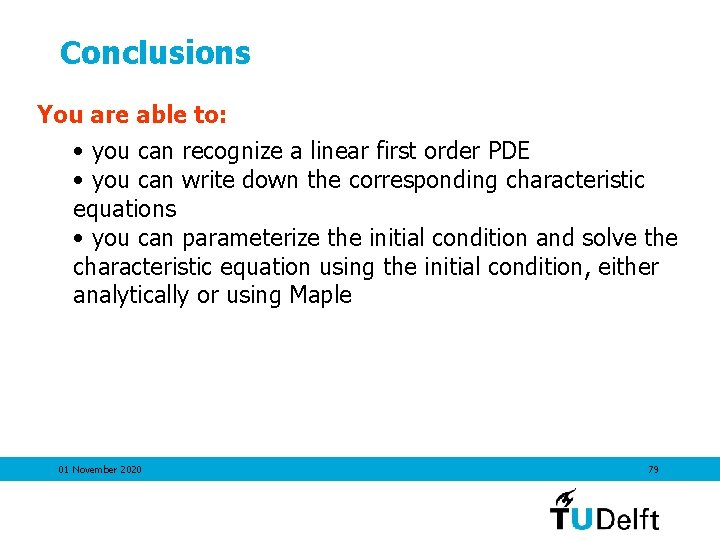Conclusions You are able to: • you can recognize a linear first order PDE • you can write down the corresponding characteristic equations • you can parameterize the initial condition and solve the characteristic equation using the initial condition, either analytically or using Maple 01 November 2020 79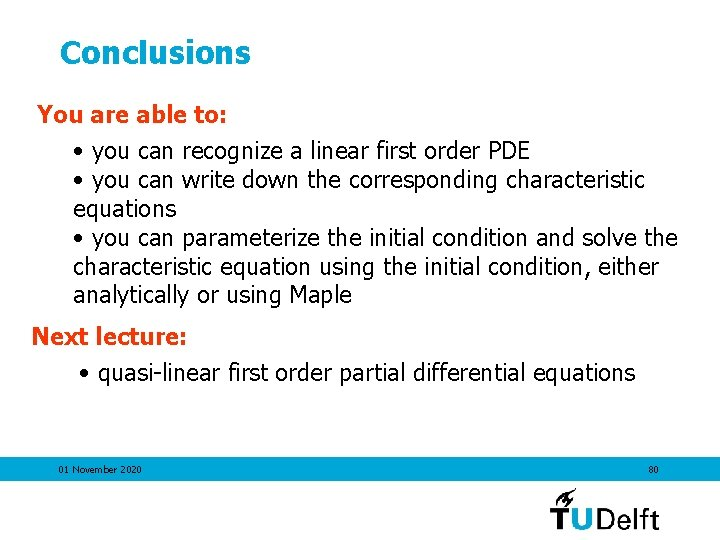Conclusions You are able to: • you can recognize a linear first order PDE • you can write down the corresponding characteristic equations • you can parameterize the initial condition and solve the characteristic equation using the initial condition, either analytically or using Maple Next lecture: • quasi-linear first order partial differential equations 01 November 2020 80